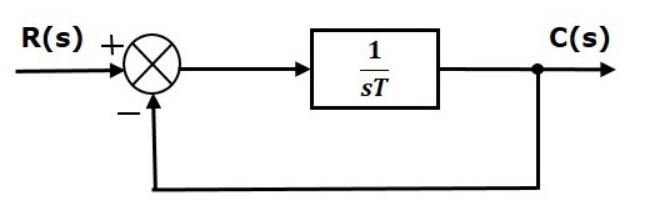### Standard 1st order System Time Response Analysis

A standard 1st order control system is given by:In a standard 1st order control system an open loop with gain is connected with a unity negative feedback loop.
The overall transfer function of the system can be  written as :

Where, G(s) is the open loop forward gain .
Substituting,

As we can se the overall power of s in denominator is still unity hence the system is of order 1.
We can rewrite the equation as:

Where,
C(s) is the laplace transform of the output signal,
R(s) is the laplace transform of the input signal,
T is the time constant
We can get the response by using the following steps:

• Take laplace transform of the input signal and get R(s).

• Substitute R(s) in the equation :

• Take inverse laplace transform to get a response in time domain.

Standard Test signals can be used as input signal to get the specific time response.